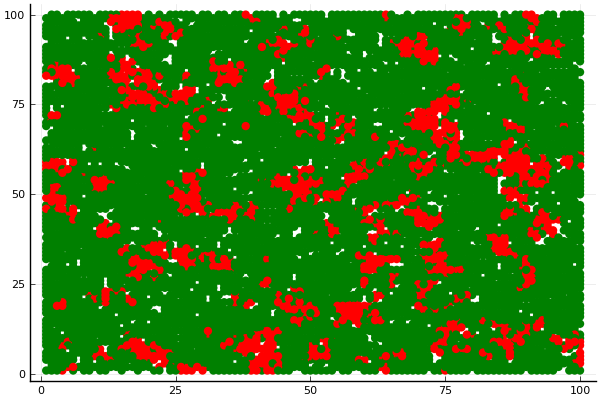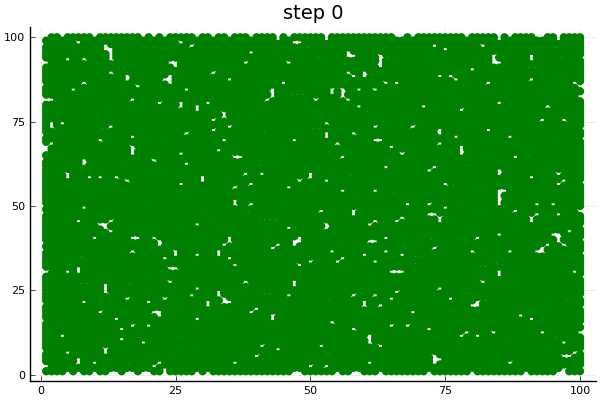# Forest fire modelThe forest fire model is defined as a cellular automaton on a grid. A cell can be empty, occupied by a tree, or burning. The model of Drossel and Schwabl (1992) is defined by four rules which are executed simultaneously:

1. A burning cell turns into an empty cell
2. A tree will burn if at least one neighbor is burning
3. A tree ignites with probability f even if no neighbor is burning
4. An empty space fills with a tree with probability p

The forest has an innate density d, which is the proportion of trees initialized as green. This model is an example that does not have an agent_step! function. It only uses a model_step!

## Defining the core structures

We start by defining the agent type

using Agents, Random, AgentsPlots

mutable struct Tree <: AbstractAgent
id::Int
pos::Tuple{Int,Int}
status::Bool  # true is green and false is burning
end

The agent type Tree has three fields: id and pos, which have to be there for any agent, and a status field that we introduce for this specific model. The status field will hold true for a green tree and false for a burning one. All other model parameters go into the AgentBasedModel.

We then make a setup function that initializes the model.

function model_initiation(; f = 0.02, d = 0.8, p = 0.01, griddims = (100, 100), seed = 111)
Random.seed!(seed)
space = GridSpace(griddims, moore = true)
properties = Dict(:f => f, :d => d, :p => p)
forest = AgentBasedModel(Tree, space; properties = properties)

# create and add trees to each node with probability d,
# which determines the density of the forest
for node in nodes(forest)
if rand() ≤ forest.d
end
end
return forest
end

forest = model_initiation(f = 0.05, d = 0.8, p = 0.05, griddims = (20, 20), seed = 2)
AgentBasedModel with 327 agents of type Tree
space: GridSpace with 400 nodes and 1482 edges
scheduler: fastest
properties: Dict(:f => 0.05,:p => 0.05,:d => 0.8)

## Defining the step!

Because of the way the forest fire model is defined, we only need a stepping function for the model

function forest_step!(forest)
for node in nodes(forest, by = :random)
nc = get_node_contents(node, forest)
# the cell is empty, maybe a tree grows here
if length(nc) == 0
rand() ≤ forest.p && add_agent!(node, forest, true)
else
tree = forest[nc] # by definition only 1 agent per node
if tree.status == false  # if it is has been burning, remove it.
kill_agent!(tree, forest)
else
if rand() ≤ forest.f  # the tree ignites spontaneously
tree.status = false
else  # if any neighbor is on fire, set this tree on fire too
for cell in node_neighbors(node, forest)
neighbors = get_node_contents(cell, forest)
length(neighbors) == 0 && continue
if any(n -> !forest.agents[n].status, neighbors)
tree.status = false
break
end
end
end
end
end
end
end

as we discussed, there is no agent_step! function here, so we will just use dummystep.

## Running the model

step!(forest, dummystep, forest_step!, 1)
forest
AgentBasedModel with 330 agents of type Tree
space: GridSpace with 400 nodes and 1482 edges
scheduler: fastest
properties: Dict(:f => 0.05,:p => 0.05,:d => 0.8)
step!(forest, dummystep, forest_step!, 10)
forest
AgentBasedModel with 94 agents of type Tree
space: GridSpace with 400 nodes and 1482 edges
scheduler: fastest
properties: Dict(:f => 0.05,:p => 0.05,:d => 0.8)

Now we can do some data collection as well using an aggregate function percentage:

forest = model_initiation(griddims = (20, 20), seed = 2)
percentage(x) = count(x) / nv(forest)

data

11 rows × 2 columns

steppercentage_status
Int64Float64
100.8175
210.675
320.35
430.08
540.035
650.03
760.0375
870.045
980.06
1090.065
11100.07

Now let's plot the model using green and red color for alive/burning

forest = model_initiation()
step!(forest, dummystep, forest_step!, 1)
treecolor(a) = a.status == 1 ? :green : :red
plotabm(forest; ac = treecolor, ms = 6, msw = 0)or animate it

forest = model_initiation(f = 0.005)
anim = @animate for i in 0:20
i > 0 && step!(forest, dummystep, forest_step!, 1)
p1 = plotabm(forest; ac = treecolor, ms = 6, msw = 0)
title!(p1, "step \$(i)")
end

gif(anim, "forest.gif", fps = 2)